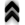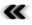### 7.1 Equilibrium and Macroscopic Quantities

Faced with severe diﬃculties of ﬁnding solutions of Boltzmann’s equation, Boltzmann himself was able to derive the shape of the equilibrium state of the distribution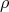in the shape of the Maxwell-Boltzmann distribution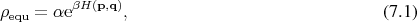where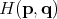is the system’s Hamiltonian,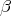is connected to the average energy and thus the temperature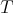and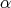a factor additionally accounting for the number of degrees of freedom.

Furthermore, Hilbert’s investigations  of the dynamics of Boltzmann’s equations using an expansion into a power series of the form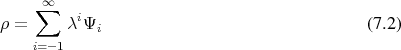revealed that in the case the collision term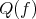, and by extension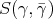, is symmetric, the evolution described by Boltzmann’s equation can be captured by using ﬁve distinct functions. These can incidentally be linked to the macroscopic quantities of density, temperature and velocity. Thus establishing that the evolution of systems described under these circumstances can be characterized using hydrodynamic descriptions .

The interconnection between the theory of Boltzmann’s description and the macroscopic transport mechanisms becomes more apparent in lieu of the theory in Section 5.5, which allows to derive a series of macroscopic quantities as well as equations connecting these quantities and governing their evolution. In this context, Boltzmann’s statement regarding the shape of the equilibrium provides a guide for admissible assumptions during further derivations, while Hilbert’s theoretical result provides a strong argument how many quantities and hence equations must be considered.

When the conditions regarding symmetry are satisﬁed, the derivation of further quantities is not only not furthering the cause of obtaining better approximations, but on the contrary introduces unwarranted complications due to the computational eﬀort which needlessly squanders resources. Thus the crunching of numbers can be reduced by employing information, which has been arrived at through completely theoretical means.

#### Macroscopic Description of Electrons in Semiconductors

While Boltzmann conducted his research in the ﬁeld of gas dynamics, his equation has found applications beyond this initial ﬁeld. One of these ﬁelds is the description of electron dynamics in semiconductors. Where the scattering term in gas dynamics mainly considers interactions between diﬀerent particles, the case of semiconductors focuses on the interaction of an electron with the surrounding semiconductor crystal. Thus, while in the ﬁeld of gas dynamics the energy contained in the gas can be considered a conserved quantity, especially if not considering any boundaries, this is no longer appropriate in the case of solid state physics, since the electrons interact with the crystal lattice and thus gain or remove energy form the considered system. This necessitates a change in the symmetry of the scattering operator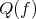1.

Since the symmetry considerations have been involved in the determination of the required number of equations, a reexamination of the previously suﬃcient set of equations is in order in this context. Thusly motivated, a ladder of equations has been derived following the scheme sketched in Section 5.5, which exceeds the previously established systems in complexity . The complexity is further increased, as any system of equations derived from Boltzmann’s equation must be solved self consistently with a coupled Poisson equation, due to the charge of the involved particle. The involvement of charge, furthermore, leads to a doubling of eﬀort, as positive and negative particles may be considered.

From among the derivable sets of equations the most widely used is also the simplest, which can also be easily derived on empirical considerations. The equations describe convection-diﬀusion, also called drift-diﬀusion, problem.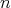is the number of electrons,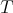the temperature,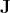the electron current,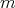and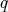are the electron mass and charge, respectively,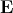is the electric ﬁeld, and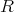a term describing recombination with holes.

This model has been in use from early days of the semiconductor industry, when computational resources were far scarcer than they are today. For this reason it was of greatest importance for the applicability of this approach to develop a discretization scheme which maintains the level of accuracy with as coarse a spatial discretization as possible. Thus the computational eﬀort has been considerably reduced, by accounting for the exponential distribution of electrons and at the same time consistently modelling the potential linearly .

Aiming to increase the accuracy of the physical description, the same discretization scheme has also been applied to the equations derived using higher powers of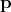. This, however, is only of limited success, as the increased computational eﬀort is additionally coupled with problems of obtaining a solution at all. Thus the more complex models have, so far, not found great adoption. While the problems encountered in dealing with higher order transport models may very well be attributed to their heightened complexity alone, it cannot be neglected that the applied discretization scheme, which mimics the highly successful Scharfetter-Gummel discretization of the drift diﬀusion model, is no longer consistent even in the case of a hydrodynamic, in this context also often called energy transport, description. Thus as the physical models of increasing complexity are additionally hampered by inconsistencies in their mapping from the continuous to the digital world, numerical diﬃculties are hardly surprising. While these may be unavoidable due to added degrees of freedom, even the most rigorous theoretical derivation of models will be greatly handicapped and impaired, when the ﬁnal translation to a discrete setting is fragmented, especially in the unforgiving world of non-linear partial diﬀerential equations.

Despite the problems encountered in further reﬁnement, the value of this approach to obtaining approximations to Boltzmann’s equation should not be neglected. Especially when considering the case of the drift-diﬀusion model, the required computational resources are comparatively modest compared to alternate methods of tackling the problem presented by Boltzmann’s equation.